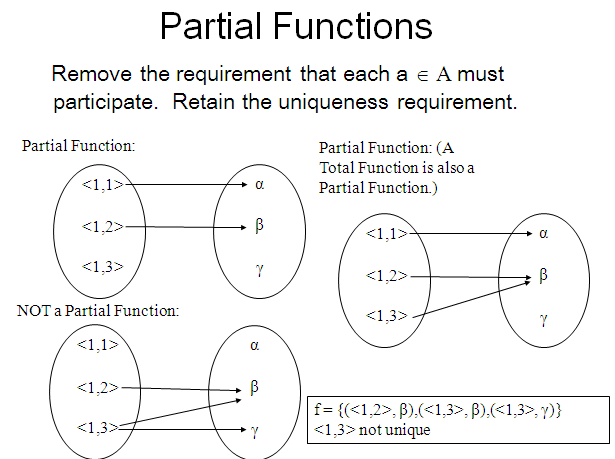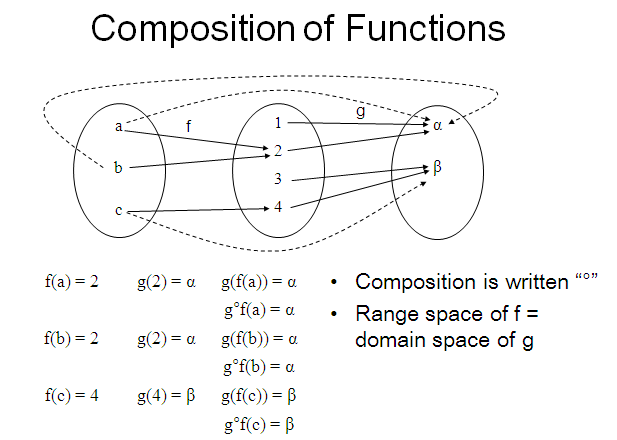# SCC Education

## function and relation,notation of function, domain and range,special function,composition of functions,injection

A function is a relation between a set of inputs and a set

of permissible outputs with the property that each input

is related to exactly one output. An example is

the function that relates each real number x to its square x2.some-important-formulas-of-integration

solving-models-related-to-ordinary differential equation

differentiation-of-exponential-functions

area-between-curve

vector

application-of-derivatives

homogenous-differential-equation

inverse-trigonometry-questions

integration

integration-questions

Rolles-theorem-and-mean-value-theorem

Techniques-of-integration-substitution

critical-points-and-point-of-inflection

intermediate-value-theorem

### Sample paper Mathematics 12